# Relating Airy and Bessel functions

The Airy functions Ai(x) and Bi(x) are independent solutions to the differential equation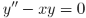For negative x they act something like sin(x) and cos(x). For positive x they act something like exp(x) and exp(-x). This isn’t surprising if you look at the differential equation. If you replace x with a negative constant, you get sines and cosines, and if you replace it with a positive constant, you get positive and negative exponentials.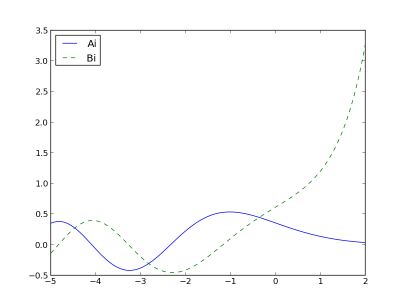The Airy functions can be related to Bessel functions as follows: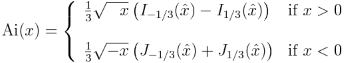and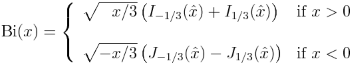Here J is a “Bessel function of the first kind” and I is a “modified Bessel function of the first kind.” Also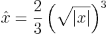To verify the equations above, and to show how to compute these functions in Python, here’s some code.

The SciPy function airy computes both functions, and their first derivatives, at once. I assume that’s because it doesn’t take much longer to compute all four functions than to compute one. The code for Ai2 and Bi2 below uses np.where instead of if … else so that it can operate on NumPy vectors all at once. You can plot Ai and Ai2 and see that the two curves lie on top of each other. The same holds for Bi and Bi2.

from scipy.special import airy, jv, iv
from numpy import sqrt, where

def Ai(x):
(ai, ai_prime, bi, bi_prime) = airy(x)
return ai

def Bi(x):
(ai, ai_prime, bi, bi_prime) = airy(x)
return bi

def Ai2(x):
third = 1.0/3.0
hatx = 2*third*(abs(x))**1.5
return where(x > 0,
third*sqrt( x)*(iv(-third, hatx) - iv(third, hatx)),
third*sqrt(-x)*(jv(-third, hatx) + jv(third, hatx)))

def Bi2(x):
third = 1.0/3.0
hatx = 2*third*(abs(x))**1.5
return where(x > 0,
sqrt( x/3.0)*(iv(-third, hatx) + iv(third, hatx)),
sqrt(-x/3.0)*(jv(-third, hatx) - jv(third, hatx)))


There is a problem with Ai2 and Bi2: they return nan at 0. A more careful implementation would avoid this problem, but that’s not necessary since these functions are only for illustration. In practice, you’d simply use airy and it does the right thing at 0.